# Normal

(diff) ← Older revision | Latest revision (diff) | Newer revision → (diff)

to a curve (or surface) at a point of it

A straight line passing through the point and perpendicular to the tangent (or tangent plane) of the curve (or surface) at this point. A smooth plane curve has at every point a unique normal situated in the plane of the curve. If a curve in a plane is given in rectangular coordinates by an equation, then the equation of the normal to the curve at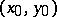has the form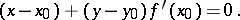A curve in space has infinitely many normals at every point of it. These fill a certain plane (the normal plane). The normal lying in the osculating plane is called the principal normal; the one perpendicular to the osculating plane is called the binormal.

The normal atto a surface given by an equationis defined by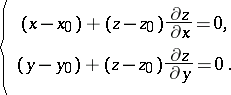If the equation of the surface has the form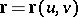, then the parametric representation of the normal isThe notion of a normal obviously extends to-dimensional submanifolds of Euclidean-space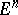, giving an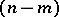-dimensional affine subspace as the normal-plane to the manifold at the corresponding point. For submanifolds of (pseudo-) Riemannian manifolds, the normal planes are considered as subspaces of the tangent space of the ambient space, where orthogonality is defined by means of the (ambient) (pseudo-) Riemannian metric. See also Normal bundle; Normal plane; Normal space (to a surface).